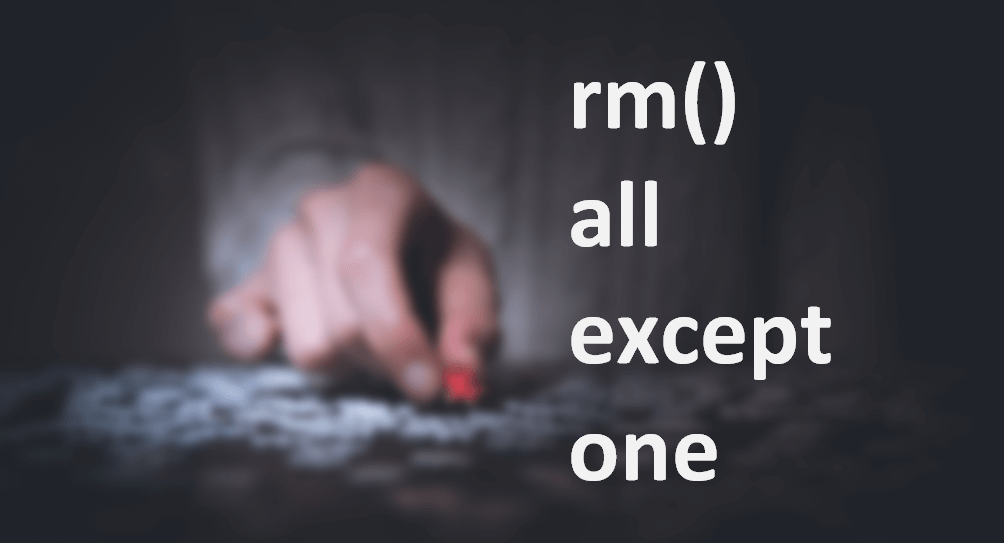# Remove all objects except one or few in RSometimes you need an almost fresh start, and, in that case, here is how to remove all objects except one or few in R.

With the function ls, you can get a list of all objects. With the function rm, you can remove specified objects.

```a <- b <- c <- d <- 1

ls()

# "a" "b" "c" "d"```

If in the list of all objects is something you would like to keep, you can detect that with function match. That position gives which value you need to remove from the list in R.

```a <- b <- c <- d <- 1

match("c", ls())

# 3```

### Remove all objects except one in R

```a <- b <- c <- d <- 1

rm(list = ls()[-match("c", ls())])

ls()

# "c"```

Here is how to do that if you have more than one object to remove from the list.

```a <- b <- c <- d <- 1

rm(list = ls()[-match(c("c", "d"), ls())])```

After removing all necessary objects, you can also clear cache in R. If you are doing that from the console, then here is a little trick on how to run multiple functions one after another. By using a semicolon in between.

```a <- b <- c <- d <- 1

rm(list = ls()[-match("c", ls())]) ; gc()```

If you would like to clear unnecessary clutter in R, then I recommend this post.

How to clear RStudio panes with code

### 2 comments on “Remove all objects except one or few in R”

1.I just spent several hours hunting for the answer to a similar but slightly different question – I needed to be able to delete all objects in R (including functions) except a handful of vectors.

•Janis Sturis

Did you successfully solved it?